# Costs and benefits of behavioral specialization

by Arne Brutschy, Nam-Luc Tran, Nadir Baiboun, Marco Frison, Giovanni Pini, Andrea Roli, Marco Dorigo, and Mauro Birattari

Last change: May 25th, 2011

## Abstract

In this work, we study behavioral specialization in a swarm of autonomous robots. In the studied swarm, a robot working repeatedly on the same type of task improves in task performance due to learning. This positive effect of learning can be exploited by the robots by behaviorally specializing on the tasks they are good at. However, even though the exploitation of such performance-improving effects is clearly a benefit, specialization also entails certain costs. Using a task allocation strategy that allows the robots to behaviorally specialize, we study the trade-off between costs and benefits in simulation experiments. Additionally, we give a perspective on the impact of this trade-off in systems that use specialization.

Keywords: specialization, task allocation, swarm robotics, swarm intelligence, self-organization, division of labor.

## Q-Q Plots of experiment 1: throughput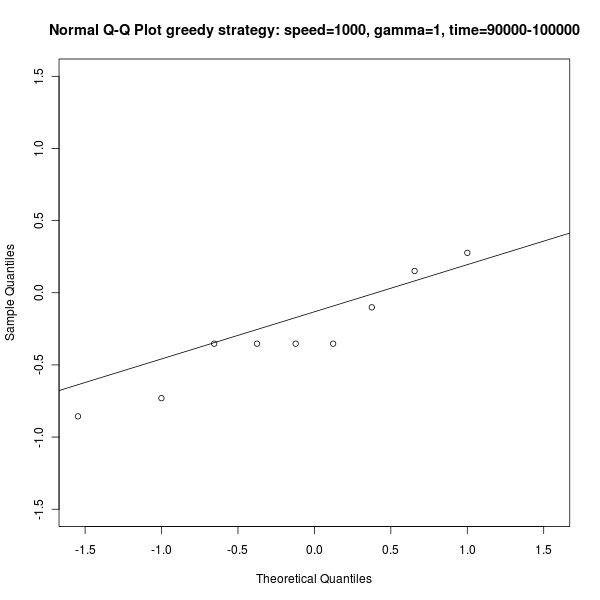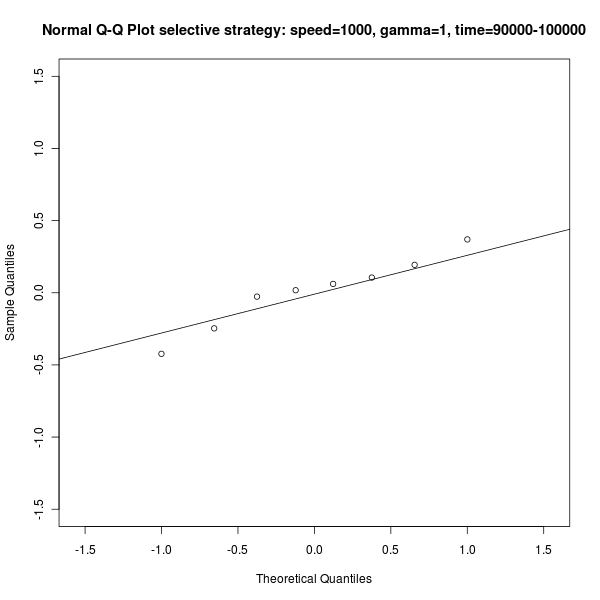Figure s1: Example Q-Q Plot for distribution of performance measured in task completed, for the last 1000 seconds of the experiment (t=9000-10000). Left: the greedy strategy. Right: The selective stategy. In both cases the performance is normally distributed.

## Statistical analysis of experiment 1: throughput

Output of the statistical analysis of the performance of the two strategies, measured as task completed in a time interval of 1000 seconds.

```Time interval 0-10000
* Distribution selective strategy *
Min. 1st Qu.  Median    Mean 3rd Qu.    Max.
58.0    60.5    62.0    61.6    63.0    64.0
mean=61.600000, sd=1.955050 (2.107148%), var=3.822222, se=0.618241 (1.003638%)
* Distribution greedy strategy *
Min. 1st Qu.  Median    Mean 3rd Qu.    Max.
56.00   59.25   61.00   60.60   61.75   65.00
mean=60.600000, sd=2.796824 (4.615220%), var=7.822222, se=0.884433 (1.459461%)
* Statistical significance *
selective strategy is non-significantly better (p=0.367765)

Time interval 10000-20000
* Distribution selective strategy *
Min. 1st Qu.  Median    Mean 3rd Qu.    Max.
73.00   75.00   76.00   76.30   77.75   81.00
mean=76.300000, sd=2.311805 (2.491658%), var=5.344444, se=0.731057 (0.958135%)
* Distribution greedy strategy *
Min. 1st Qu.  Median    Mean 3rd Qu.    Max.
65.00   66.00   67.00   67.60   68.75   72.00
mean=67.600000, sd=2.412928 (3.569420%), var=5.822222, se=0.763035 (1.128750%)
* Statistical significance *
selective strategy is significantly better (p=0.000000)

Time interval 20000-30000
* Distribution selective strategy *
Min. 1st Qu.  Median    Mean 3rd Qu.    Max.
79.0    88.5    95.0    94.6   102.0   104.0
mean=94.600000, sd=8.355969 (9.006042%), var=69.822222, se=2.642389 (2.793224%)
* Distribution greedy strategy *
Min. 1st Qu.  Median    Mean 3rd Qu.    Max.
65.00   69.25   70.00   70.20   71.50   74.00
mean=70.200000, sd=2.485514 (3.540618%), var=6.177778, se=0.785988 (1.119642%)
* Statistical significance *
selective strategy is significantly better (p=0.000003)

Time interval 30000-40000
* Distribution selective strategy *
Min. 1st Qu.  Median    Mean 3rd Qu.    Max.
91.0   108.2   111.5   110.5   115.2   119.0
mean=110.500000, sd=8.058812 (8.685766%), var=64.944444, se=2.548420 (2.306262%)
* Distribution greedy strategy *
Min. 1st Qu.  Median    Mean 3rd Qu.    Max.
68.00   69.25   70.00   70.70   72.75   74.00
mean=70.700000, sd=2.162817 (3.059147%), var=4.677778, se=0.683943 (0.967387%)
* Statistical significance *
selective strategy is significantly better (p=0.000000)

Time interval 40000-50000
* Distribution selective strategy *
Min. 1st Qu.  Median    Mean 3rd Qu.    Max.
106.0   110.8   113.5   114.4   119.5   121.0
mean=114.400000, sd=5.189733 (5.593481%), var=26.933333, se=1.641138 (1.434561%)
* Distribution greedy strategy *
Min. 1st Qu.  Median    Mean 3rd Qu.    Max.
60.00   68.00   71.00   70.50   73.75   77.00
mean=70.500000, sd=4.859127 (6.892378%), var=23.611111, se=1.536591 (2.179561%)
* Statistical significance *
selective strategy is significantly better (p=0.000000)

Time interval 50000-60000
* Distribution selective strategy *
Min. 1st Qu.  Median    Mean 3rd Qu.    Max.
106.0   113.0   114.5   114.0   116.0   120.0
mean=114.000000, sd=3.887301 (4.189723%), var=15.111111, se=1.229273 (1.078309%)
* Distribution greedy strategy *
Min. 1st Qu.  Median    Mean 3rd Qu.    Max.
68.00   69.25   72.00   73.60   78.25   81.00
mean=73.600000, sd=5.232378 (7.109209%), var=27.377778, se=1.654623 (2.248129%)
* Statistical significance *
selective strategy is significantly better (p=0.000000)

Time interval 60000-70000
* Distribution selective strategy *
Min. 1st Qu.  Median    Mean 3rd Qu.    Max.
100.0   114.5   117.0   115.9   119.5   124.0
mean=115.900000, sd=6.573516 (7.084918%), var=43.211111, se=2.078728 (1.793553%)
* Distribution greedy strategy *
Min. 1st Qu.  Median    Mean 3rd Qu.    Max.
43.00   70.25   72.50   70.40   74.00   81.00
mean=70.400000, sd=10.340857 (14.688718%), var=106.933333, se=3.270066 (4.644980%)
* Statistical significance *
selective strategy is significantly better (p=0.000000)

Time interval 70000-80000
* Distribution selective strategy *
Min. 1st Qu.  Median    Mean 3rd Qu.    Max.
102.0   107.5   115.0   113.9   118.8   124.0
mean=113.900000, sd=7.400450 (7.976186%), var=54.766667, se=2.340228 (2.054634%)
* Distribution greedy strategy *
Min. 1st Qu.  Median    Mean 3rd Qu.    Max.
68.00   75.50   78.00   76.70   78.75   82.00
mean=76.700000, sd=3.888730 (5.070052%), var=15.122222, se=1.229724 (1.603291%)
* Statistical significance *
selective strategy is significantly better (p=0.000000)

Time interval 80000-90000
* Distribution selective strategy *
Min. 1st Qu.  Median    Mean 3rd Qu.    Max.
71.0   100.2   109.0   105.8   114.8   123.0
mean=105.800000, sd=14.718092 (15.863121%), var=216.622222, se=4.654269 (4.399120%)
* Distribution greedy strategy *
Min. 1st Qu.  Median    Mean 3rd Qu.    Max.
45.00   74.00   77.00   74.60   77.75   89.00
mean=74.600000, sd=11.899580 (15.951179%), var=141.600000, se=3.762978 (5.044206%)
* Statistical significance *
selective strategy is significantly better (p=0.000067)

Time interval 90000-100000
* Distribution selective strategy *
Min. 1st Qu.  Median    Mean 3rd Qu.    Max.
66.0   109.2   114.5   113.6   117.5   160.0
mean=113.600000, sd=22.706827 (24.473359%), var=515.600000, se=7.180529 (6.320888%)
* Distribution greedy strategy *
Min. 1st Qu.  Median    Mean 3rd Qu.    Max.
74.0    78.0    78.0    80.8    81.5   102.0
mean=80.800000, sd=7.941452 (9.828530%), var=63.066667, se=2.511308 (3.108054%)
* Statistical significance *
selective strategy is significantly better (p=0.001189)
```

## Q-Q Plots of experiment 2: adaptivity, F measure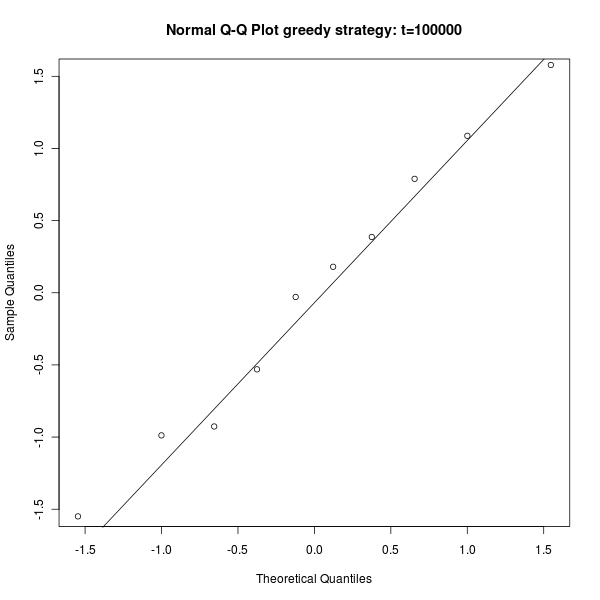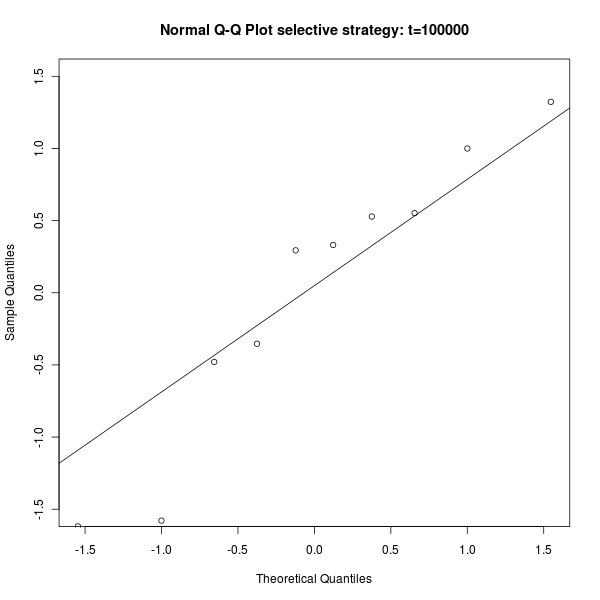Figure s2: Example Q-Q Plot for distribution of the F measure, at the end of the experiment. Left: the greedy strategy. Right: The selective stategy. The P measure is not normally distributed.

## Q-Q Plots of experiment 2: adaptivity, P measure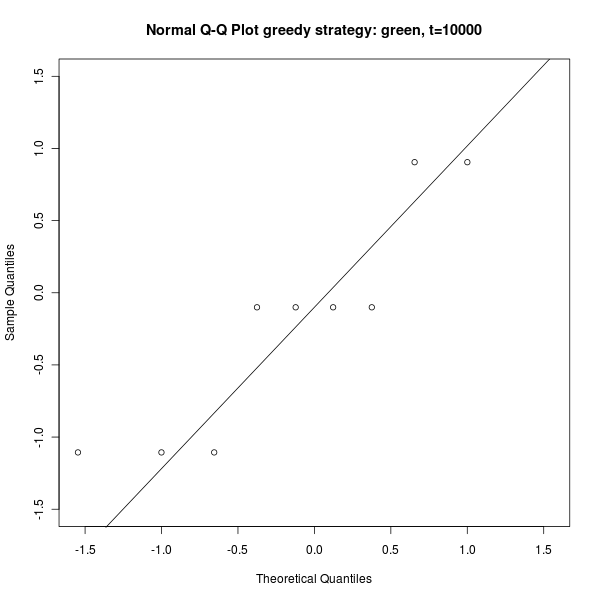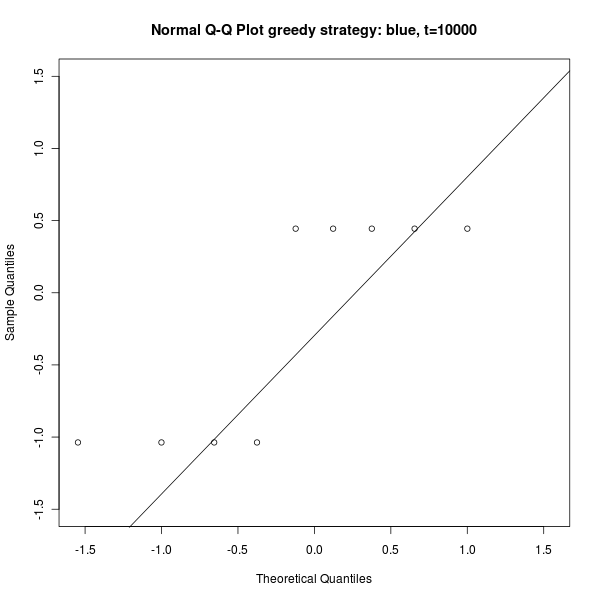Figure s3: Example Q-Q Plot for distribution of the P measure in case of the selective strategy, at the end of the experiment. Left: P measure for the green tasks. Right: P measure for the blue tasks. The P measure is not normally distributed.

## Q-Q Plots of experiment 3: cost and benefits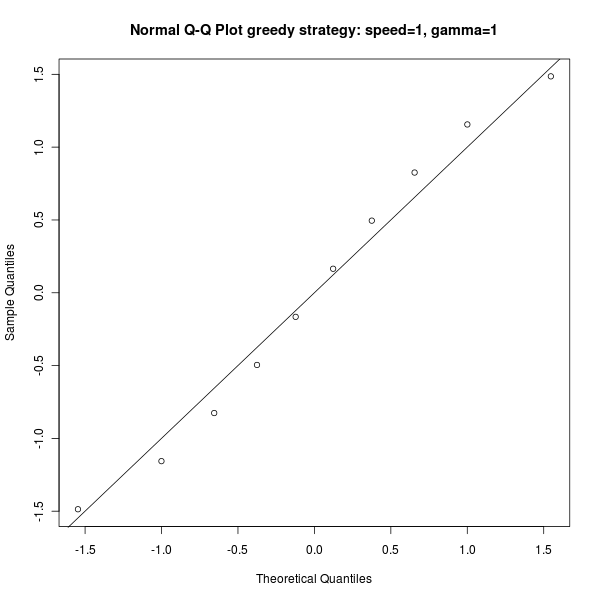Figure s4: Example Q-Q Plot for distribution of performance measured in task completed, for different settings of the cost and benefit tradeoff (search time vs. task completion time), at the end of the experiment. Left: the greedy strategy. Right: The selective stategy. In both cases the performance is normally distributed.

## Statistical analysis of experiment 3: cost and benefits

Output of the statistical analysis of the performance of the two strategies, for different settings of the cost and benefit tradeoff (search time vs. task completion time).

```Experimental set speed=100, gamma=1:
* Distribution of selective strategy *
Min. 1st Qu.  Median    Mean 3rd Qu.    Max.
733.0   739.5   757.5   760.8   781.8   797.0
mean=760.800000, sd=24.229459 (3.184734%), var=587.066667, se=7.662028 (1.007101%)
* Distribution of greedy stratgey *
Min. 1st Qu.  Median    Mean 3rd Qu.    Max.
610.0   620.0   628.0   628.2   634.0   647.0
mean=628.200000, sd=11.839670 (1.884697%), var=140.177778, se=3.744032 (0.595994%)
* Significance *
selective strategy is significantly better (p=0.000000)

Experimental set speed=100, gamma=2:
* Distribution of selective strategy *
Min. 1st Qu.  Median    Mean 3rd Qu.    Max.
639.0   681.5   701.5   695.4   711.8   727.0
mean=695.400000, sd=26.132993 (3.757980%), var=682.933333, se=8.263978 (1.188378%)
* Distribution of greedy stratgey *
Min. 1st Qu.  Median    Mean 3rd Qu.    Max.
593.0   598.5   608.0   611.3   622.0   639.0
mean=611.300000, sd=16.028100 (2.621970%), var=256.900000, se=5.068530 (0.829140%)
* Significance *
selective strategy is significantly better (p=0.000000)

Experimental set speed=100, gamma=3:
* Distribution of selective strategy *
Min. 1st Qu.  Median    Mean 3rd Qu.    Max.
604.0   622.8   632.0   630.2   641.0   643.0
mean=630.200000, sd=12.804513 (2.031817%), var=163.955556, se=4.049143 (0.642517%)
* Distribution of greedy stratgey *
Min. 1st Qu.  Median    Mean 3rd Qu.    Max.
591.0   602.8   610.0   610.1   615.0   635.0
mean=610.100000, sd=12.114729 (1.985696%), var=146.766667, se=3.831014 (0.627932%)
* Significance *
selective strategy is significantly better (p=0.002029)

Experimental set speed=100, gamma=4:
* Distribution of selective strategy *
Min. 1st Qu.  Median    Mean 3rd Qu.    Max.
519.0   547.5   560.0   556.8   571.0   582.0
mean=556.800000, sd=21.311447 (3.827487%), var=454.177778, se=6.739271 (1.210358%)
* Distribution of greedy stratgey *
Min. 1st Qu.  Median    Mean 3rd Qu.    Max.
581.0   586.8   591.5   591.8   596.2   604.0
mean=591.800000, sd=7.208020 (1.217982%), var=51.955556, se=2.279376 (0.385160%)
* Significance *
selective strategy is significantly worse (p=0.000453)

Experimental set speed=200, gamma=1:
* Distribution of selective strategy *
Min. 1st Qu.  Median    Mean 3rd Qu.    Max.
832.0   843.0   858.0   857.5   865.0   892.0
mean=857.500000, sd=20.194884 (2.355088%), var=407.833333, se=6.386183 (0.744744%)
* Distribution of greedy stratgey *
Min. 1st Qu.  Median    Mean 3rd Qu.    Max.
659.0   662.0   666.0   667.4   672.0   680.0
mean=667.400000, sd=7.574812 (1.134973%), var=57.377778, se=2.395366 (0.358910%)
* Significance *
selective strategy is significantly better (p=0.000000)

Experimental set speed=200, gamma=2:
* Distribution of selective strategy *
Min. 1st Qu.  Median    Mean 3rd Qu.    Max.
696.0   757.0   761.5   761.9   772.8   803.0
mean=761.900000, sd=30.200993 (3.963905%), var=912.100000, se=9.550393 (1.253497%)
* Distribution of greedy stratgey *
Min. 1st Qu.  Median    Mean 3rd Qu.    Max.
632.0   642.0   644.0   645.6   649.0   668.0
mean=645.600000, sd=9.731963 (1.507429%), var=94.711111, se=3.077517 (0.476691%)
* Significance *
selective strategy is significantly better (p=0.000000)

Experimental set speed=200, gamma=3:
* Distribution of selective strategy *
Min. 1st Qu.  Median    Mean 3rd Qu.    Max.
629.0   674.5   684.0   679.5   696.0   711.0
mean=679.500000, sd=26.175688 (3.852198%), var=685.166667, se=8.277479 (1.218172%)
* Distribution of greedy stratgey *
Min. 1st Qu.  Median    Mean 3rd Qu.    Max.
620.0   631.0   637.5   637.0   645.2   651.0
mean=637.000000, sd=9.944289 (1.561113%), var=98.888889, se=3.144660 (0.493667%)
* Significance *
selective strategy is significantly better (p=0.000483)

Experimental set speed=200, gamma=4:
* Distribution of selective strategy *
Min. 1st Qu.  Median    Mean 3rd Qu.    Max.
561.0   614.8   618.5   611.0   626.5   637.0
mean=611.000000, sd=25.944385 (4.246217%), var=673.111111, se=8.204335 (1.342772%)
* Distribution of greedy stratgey *
Min. 1st Qu.  Median    Mean 3rd Qu.    Max.
602.0   623.0   628.0   625.5   629.0   640.0
mean=625.500000, sd=9.991663 (1.597388%), var=99.833333, se=3.159641 (0.505139%)
* Significance *
selective strategy is non-significantly worse (p=0.125857)

Experimental set speed=300, gamma=1:
* Distribution of selective strategy *
Min. 1st Qu.  Median    Mean 3rd Qu.    Max.
870.0   910.2   917.0   915.7   927.8   942.0
mean=915.700000, sd=20.688429 (2.259302%), var=428.011111, se=6.542256 (0.714454%)
* Distribution of greedy stratgey *
Min. 1st Qu.  Median    Mean 3rd Qu.    Max.
637.0   674.2   679.5   678.0   693.0   696.0
mean=678.000000, sd=18.312109 (2.700901%), var=335.333333, se=5.790797 (0.854100%)
* Significance *
selective strategy is significantly better (p=0.000000)

Experimental set speed=300, gamma=2:
* Distribution of selective strategy *
Min. 1st Qu.  Median    Mean 3rd Qu.    Max.
732.0   784.2   789.0   787.7   802.8   819.0
mean=787.700000, sd=26.183328 (3.324023%), var=685.566667, se=8.279895 (1.051148%)
* Distribution of greedy stratgey *
Min. 1st Qu.  Median    Mean 3rd Qu.    Max.
640.0   652.0   661.0   660.1   666.2   685.0
mean=660.100000, sd=13.059267 (1.978377%), var=170.544444, se=4.129703 (0.625618%)
* Significance *
selective strategy is significantly better (p=0.000000)

Experimental set speed=300, gamma=3:
* Distribution of selective strategy *
Min. 1st Qu.  Median    Mean 3rd Qu.    Max.
647.0   710.5   728.0   717.0   735.5   748.0
mean=717.000000, sd=30.565049 (4.262908%), var=934.222222, se=9.665517 (1.348050%)
* Distribution of greedy stratgey *
Min. 1st Qu.  Median    Mean 3rd Qu.    Max.
633.0   643.8   658.5   653.1   663.8   666.0
mean=653.100000, sd=12.982467 (1.987822%), var=168.544444, se=4.105416 (0.628605%)
* Significance *
selective strategy is significantly better (p=0.000052)

Experimental set speed=300, gamma=4:
* Distribution of selective strategy *
Min. 1st Qu.  Median    Mean 3rd Qu.    Max.
563.0   633.2   639.5   636.5   652.8   662.0
mean=636.500000, sd=28.068369 (4.409799%), var=787.833333, se=8.875998 (1.394501%)
* Distribution of greedy stratgey *
Min. 1st Qu.  Median    Mean 3rd Qu.    Max.
609.0   624.5   637.0   634.7   642.5   663.0
mean=634.700000, sd=15.627966 (2.462260%), var=244.233333, se=4.941997 (0.778635%)
* Significance *
selective strategy is non-significantly better (p=0.861887)

Experimental set speed=400, gamma=1:
* Distribution of selective strategy *
Min. 1st Qu.  Median    Mean 3rd Qu.    Max.
816.0   906.0   932.5   924.5   956.2   989.0
mean=924.500000, sd=54.691559 (5.915799%), var=2991.166667, se=17.294990 (1.870740%)
* Distribution of greedy stratgey *
Min. 1st Qu.  Median    Mean 3rd Qu.    Max.
671.0   692.8   705.5   701.0   711.2   723.0
mean=701.000000, sd=16.316318 (2.327577%), var=266.222222, se=5.159673 (0.736045%)
* Significance *
selective strategy is significantly better (p=0.000000)

Experimental set speed=400, gamma=2:
* Distribution of selective strategy *
Min. 1st Qu.  Median    Mean 3rd Qu.    Max.
717.0   814.0   830.0   818.1   837.0   850.0
mean=818.100000, sd=38.871441 (4.751429%), var=1510.988889, se=12.292229 (1.502534%)
* Distribution of greedy stratgey *
Min. 1st Qu.  Median    Mean 3rd Qu.    Max.
653.0   678.8   683.5   684.8   694.2   703.0
mean=684.800000, sd=14.815907 (2.163538%), var=219.511111, se=4.685201 (0.684171%)
* Significance *
selective strategy is significantly better (p=0.000000)

Experimental set speed=400, gamma=3:
* Distribution of selective strategy *
Min. 1st Qu.  Median    Mean 3rd Qu.    Max.
656.0   714.5   733.5   725.1   740.8   752.0
mean=725.100000, sd=28.512960 (3.932280%), var=812.988889, se=9.016590 (1.243496%)
* Distribution of greedy stratgey *
Min. 1st Qu.  Median    Mean 3rd Qu.    Max.
653.0   661.5   667.0   667.0   674.5   680.0
mean=667.000000, sd=9.006171 (1.350250%), var=81.111111, se=2.848001 (0.426987%)
* Significance *
selective strategy is significantly better (p=0.000079)

Experimental set speed=400, gamma=4:
* Distribution of selective strategy *
Min. 1st Qu.  Median    Mean 3rd Qu.    Max.
618.0   647.8   660.5   655.4   671.5   674.0
mean=655.400000, sd=20.145030 (3.073700%), var=405.822222, se=6.370418 (0.971989%)
* Distribution of greedy stratgey *
Min. 1st Qu.  Median    Mean 3rd Qu.    Max.
644.0   652.2   653.0   654.7   660.0   662.0
mean=654.700000, sd=5.812821 (0.887860%), var=33.788889, se=1.838175 (0.280766%)
* Significance *
selective strategy is non-significantly better (p=0.917911)

Experimental set speed=500, gamma=1:
* Distribution of selective strategy *
Min. 1st Qu.  Median    Mean 3rd Qu.    Max.
933.0   963.0   974.5   976.2   992.2  1011.0
mean=976.200000, sd=22.699976 (2.325341%), var=515.288889, se=7.178363 (0.735337%)
* Distribution of greedy stratgey *
Min. 1st Qu.  Median    Mean 3rd Qu.    Max.
677.0   681.8   690.0   690.5   699.2   706.0
mean=690.500000, sd=10.352133 (1.499223%), var=107.166667, se=3.273632 (0.474096%)
* Significance *
selective strategy is significantly better (p=0.000000)

Experimental set speed=500, gamma=2:
* Distribution of selective strategy *
Min. 1st Qu.  Median    Mean 3rd Qu.    Max.
710.0   821.5   844.0   823.9   857.2   881.0
mean=823.900000, sd=57.202273 (6.942866%), var=3272.100000, se=18.088947 (2.195527%)
* Distribution of greedy stratgey *
Min. 1st Qu.  Median    Mean 3rd Qu.    Max.
666.0   670.2   673.0   676.8   683.8   693.0
mean=676.800000, sd=9.647107 (1.425400%), var=93.066667, se=3.050683 (0.450751%)
* Significance *
selective strategy is significantly better (p=0.000016)

Experimental set speed=500, gamma=3:
* Distribution of selective strategy *
Min. 1st Qu.  Median    Mean 3rd Qu.    Max.
671.0   747.2   757.0   745.4   762.2   771.0
mean=745.400000, sd=30.977411 (4.155810%), var=959.600000, se=9.795918 (1.314183%)
* Distribution of greedy stratgey *
Min. 1st Qu.  Median    Mean 3rd Qu.    Max.
643.0   649.0   665.0   662.0   669.8   680.0
mean=662.000000, sd=13.678856 (2.066293%), var=187.111111, se=4.325634 (0.653419%)
* Significance *
selective strategy is significantly better (p=0.000004)

Experimental set speed=500, gamma=4:
* Distribution of selective strategy *
Min. 1st Qu.  Median    Mean 3rd Qu.    Max.
607.0   650.5   657.0   654.8   669.5   679.0
mean=654.800000, sd=21.724538 (3.317736%), var=471.955556, se=6.869902 (1.049160%)
* Distribution of greedy stratgey *
Min. 1st Qu.  Median    Mean 3rd Qu.    Max.
629.0   655.5   658.5   657.7   664.5   669.0
mean=657.700000, sd=11.382735 (1.730688%), var=129.566667, se=3.599537 (0.547292%)
* Significance *
selective strategy is non-significantly worse (p=0.714235)

Experimental set speed=600, gamma=1:
* Distribution of selective strategy *
Min. 1st Qu.  Median    Mean 3rd Qu.    Max.
839.0   973.2   980.0   962.8   986.5   999.0
mean=962.800000, sd=49.107139 (5.100451%), var=2411.511111, se=15.529041 (1.612904%)
* Distribution of greedy stratgey *
Min. 1st Qu.  Median    Mean 3rd Qu.    Max.
674.0   687.5   693.0   696.0   707.2   724.0
mean=696.000000, sd=15.084945 (2.167377%), var=227.555556, se=4.770278 (0.685385%)
* Significance *
selective strategy is significantly better (p=0.000000)

Experimental set speed=600, gamma=2:
* Distribution of selective strategy *
Min. 1st Qu.  Median    Mean 3rd Qu.    Max.
794.0   835.0   851.5   845.3   860.8   872.0
mean=845.300000, sd=22.862147 (2.704619%), var=522.677778, se=7.229646 (0.855276%)
* Distribution of greedy stratgey *
Min. 1st Qu.  Median    Mean 3rd Qu.    Max.
661.0   677.0   680.0   680.1   685.5   694.0
mean=680.100000, sd=9.194805 (1.351978%), var=84.544444, se=2.907653 (0.427533%)
* Significance *
selective strategy is significantly better (p=0.000000)

Experimental set speed=600, gamma=3:
* Distribution of selective strategy *
Min. 1st Qu.  Median    Mean 3rd Qu.    Max.
652.0   720.0   744.5   729.6   749.0   772.0
mean=729.600000, sd=36.752022 (5.037284%), var=1350.711111, se=11.622010 (1.592929%)
* Distribution of greedy stratgey *
Min. 1st Qu.  Median    Mean 3rd Qu.    Max.
646.0   665.2   668.5   667.5   673.0   687.0
mean=667.500000, sd=11.796892 (1.767325%), var=139.166667, se=3.730505 (0.558877%)
* Significance *
selective strategy is significantly better (p=0.000368)

Experimental set speed=600, gamma=4:
* Distribution of selective strategy *
Min. 1st Qu.  Median    Mean 3rd Qu.    Max.
636.0   664.0   675.5   671.2   678.8   696.0
mean=671.200000, sd=17.668553 (2.632383%), var=312.177778, se=5.587287 (0.832433%)
* Distribution of greedy stratgey *
Min. 1st Qu.  Median    Mean 3rd Qu.    Max.
639.0   652.2   657.0   655.3   657.8   670.0
mean=655.300000, sd=7.958922 (1.214546%), var=63.344444, se=2.516832 (0.384073%)
* Significance *
selective strategy is non-significantly better (p=0.022806)

Experimental set speed=700, gamma=1:
* Distribution of selective strategy *
Min. 1st Qu.  Median    Mean 3rd Qu.    Max.
833.0   971.0   988.0   970.5  1005.0  1030.0
mean=970.500000, sd=59.337547 (6.114121%), var=3520.944444, se=18.764180 (1.933455%)
* Distribution of greedy stratgey *
Min. 1st Qu.  Median    Mean 3rd Qu.    Max.
674.0   691.5   705.5   707.1   717.2   744.0
mean=707.100000, sd=21.005026 (2.970588%), var=441.211111, se=6.642372 (0.939382%)
* Significance *
selective strategy is significantly better (p=0.000000)

Experimental set speed=700, gamma=2:
* Distribution of selective strategy *
Min. 1st Qu.  Median    Mean 3rd Qu.    Max.
851.0   863.0   869.5   870.9   881.8   890.0
mean=870.900000, sd=13.403565 (1.539048%), var=179.655556, se=4.238579 (0.486690%)
* Distribution of greedy stratgey *
Min. 1st Qu.  Median    Mean 3rd Qu.    Max.
671.0   684.5   690.0   689.7   696.8   710.0
mean=689.700000, sd=12.111060 (1.755990%), var=146.677778, se=3.829853 (0.555293%)
* Significance *
selective strategy is significantly better (p=0.000000)

Experimental set speed=700, gamma=3:
* Distribution of selective strategy *
Min. 1st Qu.  Median    Mean 3rd Qu.    Max.
722.0   739.8   754.5   754.6   771.8   794.0
mean=754.600000, sd=23.523983 (3.117411%), var=553.377778, se=7.438937 (0.985812%)
* Distribution of greedy stratgey *
Min. 1st Qu.  Median    Mean 3rd Qu.    Max.
658.0   670.2   677.5   677.7   687.5   694.0
mean=677.700000, sd=11.795008 (1.740447%), var=139.122222, se=3.729909 (0.550378%)
* Significance *
selective strategy is significantly better (p=0.000000)

Experimental set speed=700, gamma=4:
* Distribution of selective strategy *
Min. 1st Qu.  Median    Mean 3rd Qu.    Max.
620.0   662.2   678.5   675.0   697.5   720.0
mean=675.000000, sd=31.404175 (4.652470%), var=986.222222, se=9.930872 (1.471240%)
* Distribution of greedy stratgey *
Min. 1st Qu.  Median    Mean 3rd Qu.    Max.
633.0   656.2   662.5   660.2   666.8   679.0
mean=660.200000, sd=12.479316 (1.890233%), var=155.733333, se=3.946306 (0.597744%)
* Significance *
selective strategy is non-significantly better (p=0.191747)

Experimental set speed=800, gamma=1:
* Distribution of selective strategy *
Min. 1st Qu.  Median    Mean 3rd Qu.    Max.
906.0   992.2  1022.0  1009.0  1032.0  1061.0
mean=1009.100000, sd=42.958507 (4.257111%), var=1845.433333, se=13.584673 (1.346217%)
* Distribution of greedy stratgey *
Min. 1st Qu.  Median    Mean 3rd Qu.    Max.
689.0   701.8   711.0   708.2   715.0   720.0
mean=708.200000, sd=9.975526 (1.408575%), var=99.511111, se=3.154538 (0.445430%)
* Significance *
selective strategy is significantly better (p=0.000000)

Experimental set speed=800, gamma=2:
* Distribution of selective strategy *
Min. 1st Qu.  Median    Mean 3rd Qu.    Max.
846.0   866.0   871.0   874.3   885.8   901.0
mean=874.300000, sd=16.384613 (1.874026%), var=268.455556, se=5.181270 (0.592619%)
* Distribution of greedy stratgey *
Min. 1st Qu.  Median    Mean 3rd Qu.    Max.
693.0   695.5   700.5   702.6   705.5   725.0
mean=702.600000, sd=9.979980 (1.420436%), var=99.600000, se=3.155947 (0.449181%)
* Significance *
selective strategy is significantly better (p=0.000000)

Experimental set speed=800, gamma=3:
* Distribution of selective strategy *
Min. 1st Qu.  Median    Mean 3rd Qu.    Max.
733.0   763.5   769.0   770.0   779.2   792.0
mean=770.000000, sd=16.633300 (2.160169%), var=276.666667, se=5.259911 (0.683105%)
* Distribution of greedy stratgey *
Min. 1st Qu.  Median    Mean 3rd Qu.    Max.
664.0   679.2   684.5   685.8   694.2   704.0
mean=685.800000, sd=11.867790 (1.730503%), var=140.844444, se=3.752925 (0.547233%)
* Significance *
selective strategy is significantly better (p=0.000000)

Experimental set speed=800, gamma=4:
* Distribution of selective strategy *
Min. 1st Qu.  Median    Mean 3rd Qu.    Max.
666.0   677.5   692.5   691.1   702.5   720.0
mean=691.100000, sd=16.934186 (2.450324%), var=286.766667, se=5.355060 (0.774860%)
* Distribution of greedy stratgey *
Min. 1st Qu.  Median    Mean 3rd Qu.    Max.
648.0   663.8   667.5   665.9   670.2   679.0
mean=665.900000, sd=8.517303 (1.279066%), var=72.544444, se=2.693408 (0.404476%)
* Significance *
selective strategy is significantly better (p=0.000987)

Experimental set speed=900, gamma=1:
* Distribution of selective strategy *
Min. 1st Qu.  Median    Mean 3rd Qu.    Max.
949.0   992.2  1042.0  1024.0  1054.0  1060.0
mean=1023.900000, sd=38.931421 (3.802268%), var=1515.655556, se=12.311196 (1.202383%)
* Distribution of greedy stratgey *
Min. 1st Qu.  Median    Mean 3rd Qu.    Max.
686.0   691.8   704.0   709.5   730.5   739.0
mean=709.500000, sd=21.350254 (3.009197%), var=455.833333, se=6.751543 (0.951592%)
* Significance *
selective strategy is significantly better (p=0.000000)

Experimental set speed=900, gamma=2:
* Distribution of selective strategy *
Min. 1st Qu.  Median    Mean 3rd Qu.    Max.
854.0   873.5   894.0   889.7   901.0   928.0
mean=889.700000, sd=22.939776 (2.578372%), var=526.233333, se=7.254194 (0.815353%)
* Distribution of greedy stratgey *
Min. 1st Qu.  Median    Mean 3rd Qu.    Max.
678.0   695.8   699.0   698.1   703.8   709.0
mean=698.100000, sd=8.556349 (1.225662%), var=73.211111, se=2.705755 (0.387588%)
* Significance *
selective strategy is significantly better (p=0.000000)

Experimental set speed=900, gamma=3:
* Distribution of selective strategy *
Min. 1st Qu.  Median    Mean 3rd Qu.    Max.
733.0   774.2   791.0   783.5   800.8   805.0
mean=783.500000, sd=23.100024 (2.948312%), var=533.611111, se=7.304869 (0.932338%)
* Distribution of greedy stratgey *
Min. 1st Qu.  Median    Mean 3rd Qu.    Max.
668.0   681.8   687.5   685.3   690.8   693.0
mean=685.300000, sd=7.484057 (1.092085%), var=56.011111, se=2.366667 (0.345348%)
* Significance *
selective strategy is significantly better (p=0.000000)

Experimental set speed=900, gamma=4:
* Distribution of selective strategy *
Min. 1st Qu.  Median    Mean 3rd Qu.    Max.
665.0   687.0   692.5   694.0   702.5   718.0
mean=694.000000, sd=14.583095 (2.101311%), var=212.666667, se=4.611580 (0.664493%)
* Distribution of greedy stratgey *
Min. 1st Qu.  Median    Mean 3rd Qu.    Max.
640.0   658.2   664.5   663.5   673.0   679.0
mean=663.500000, sd=12.140383 (1.829749%), var=147.388889, se=3.839126 (0.578617%)
* Significance *
selective strategy is significantly better (p=0.000086)

Experimental set speed=1000, gamma=1:
* Distribution of selective strategy *
Min. 1st Qu.  Median    Mean 3rd Qu.    Max.
962    1014    1034    1027    1041    1058
mean=1027.200000, sd=28.529906 (2.777444%), var=813.955556, se=9.021949 (0.878305%)
* Distribution of greedy stratgey *
Min. 1st Qu.  Median    Mean 3rd Qu.    Max.
692.0   708.5   718.0   719.5   723.8   756.0
mean=719.500000, sd=18.130698 (2.519902%), var=328.722222, se=5.733430 (0.796863%)
* Significance *
selective strategy is significantly better (p=0.000000)

Experimental set speed=1000, gamma=2:
* Distribution of selective strategy *
Min. 1st Qu.  Median    Mean 3rd Qu.    Max.
749.0   862.5   890.0   878.7   917.2   926.0
mean=878.700000, sd=53.738151 (6.115643%), var=2887.788889, se=16.993495 (1.933936%)
* Distribution of greedy stratgey *
Min. 1st Qu.  Median    Mean 3rd Qu.    Max.
669.0   687.5   702.0   696.5   706.0   712.0
mean=696.500000, sd=13.689006 (1.965399%), var=187.388889, se=4.328844 (0.621514%)
* Significance *
selective strategy is significantly better (p=0.000001)

Experimental set speed=1000, gamma=3:
* Distribution of selective strategy *
Min. 1st Qu.  Median    Mean 3rd Qu.    Max.
753.0   772.8   780.0   783.9   799.0   813.0
mean=783.900000, sd=20.234459 (2.581255%), var=409.433333, se=6.398698 (0.816265%)
* Distribution of greedy stratgey *
Min. 1st Qu.  Median    Mean 3rd Qu.    Max.
671.0   686.5   691.0   692.1   700.2   714.0
mean=692.100000, sd=12.395788 (1.791040%), var=153.655556, se=3.919892 (0.566377%)
* Significance *
selective strategy is significantly better (p=0.000000)

Experimental set speed=1000, gamma=4:
* Distribution of selective strategy *
Min. 1st Qu.  Median    Mean 3rd Qu.    Max.
679.0   691.5   703.5   702.7   713.2   730.0
mean=702.700000, sd=15.362653 (2.186232%), var=236.011111, se=4.858097 (0.691347%)
* Distribution of greedy stratgey *
Min. 1st Qu.  Median    Mean 3rd Qu.    Max.
643.0   659.0   669.5   666.9   673.0   682.0
mean=666.900000, sd=12.050357 (1.806921%), var=145.211111, se=3.810658 (0.571399%)
* Significance *
selective strategy is significantly better (p=0.000021)
```